Bitcoin Forum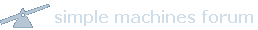January 28, 2023, 01:38:09 AM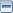News: Latest Bitcoin Core release: 24.0.1 [Torrent]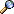Home Help Search Login Register More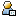Show Posts Pages: 
 2 Other / Beginners & Help / Re: How to protect B from this relatively largest miner attack? on: June 15, 2011, 11:50:05 PM Forp, I think you may have some misunderstandings of how things work. You may be confused on what a transaction is versus what a block is, or some other misunderstanding that makes your scenario really hard to understand, even to the point of making it hard to understand where the misunderstanding lies.Maybe if you can break it down into smaller parts, it would be easier to tell where the disconnect is between what you are trying to ask and what us readers see.
 3 Bitcoin / Development & Technical Discussion / Re: Mining logic basics... on: June 15, 2011, 11:35:03 PM I believe that those are mining *pool* clients, and mining pools have a lower difficulty than the actual mining difficulty [quite possible that the mining *pool* difficulty is just the top 32 bits are 0].Mining pools use these lower difficulty targets to show that their miners are doing work and then use this much smaller list of possible solutions to calculate if they also match Bitcoin's target difficulty.
 4 Bitcoin / Development & Technical Discussion / Re: How to calculate the target from getwork function? on: June 08, 2011, 10:59:39 AM Here's the current target at Block Explorer: http://blockexplorer.com/q/hextargetCode:0000000000001D932F0000000000000000000000000000000000000000000000So it looks like it is Little Endian, and if you operate on Big Endian data, you'll want to reverse it, byte by byte.
 5 Bitcoin / Development & Technical Discussion / Re: Do I understand header hashing? on: June 07, 2011, 10:01:08 PM Quote from: Enochian on June 07, 2011, 07:03:34 PMBecause you can keep resetting it when anything else in the header changes, and in any case, at least once a second.How often do you have to update the timestamp [and reset your nonce iteration]? Is it every second, such as the above implies, or are the timestamp tolerances broader than that?
 6 Bitcoin / Development & Technical Discussion / Re: Small optimizations in computing hash(hash(header)) on: June 07, 2011, 09:18:20 AM Cool.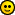I obviously had not made it to that source code yet.
 7 Bitcoin / Development & Technical Discussion / Re: Trying to understand the getwork API function on: June 07, 2011, 06:20:40 AM The value:Code:data=000000013cb5d00bd73e716c2d3c558a52b8c79fe8532b12307a7d91000008ba0000000007b7757adedda569a66c17a870bce5135faa2b3e5f46d22bed6e15d79296178f4ded6d461a1d932f00000000000000800000000000000000000000000000000000000000000000000000000000000000000000000000000080020000is Little-Endian, I believe, so the last 4 bytes are actually 0x 00 00 02 80 = 640.The leading 1 followed by padding 0s part of the SHA-256 expansion to 64 Bytes is:Code:80000000000000000000000000000000000000000000000000000000000000000000000000
8  Bitcoin / Development & Technical Discussion / Small optimizations in computing hash(hash(header)) on: June 07, 2011, 04:48:28 AM
I'll be referring to the implementation laid out in https://github.com/jgarzik/cpuminer/blob/master/sha256_generic.c in this post.

The sha256_transform is called twice per "Nonce" value. The first time on the second chunk of the header:

 Field Size (Bytes) Merkle root (last 4 Bytes) 4 Timestamp 4 "Bits" 4 Nonce 4 SHA-256 Defined bits to make the chunk 64 Bytes total 48

And then again on the resulting hash:

 Field Size (Bytes) Final hash of chunks 1 and 2 32 SHA-256 Defined bits to make the chunk 64 Bytes total 32

Due to the nature of the input we are always using [the specific BitCoin header format] and the nature of the result we are looking for [whether the final hash is smaller than the target difficulty], we can cut out some of the calculations in sha256_transform in each of those two calls.

For the first sha256_transform call [on the 2nd chunk of the header], there are several values at the beginning that can be calculated on the first "Nonce" value, and then cached for all future "Nonce" values, as long as the other fields in that chunk do not change [I understand that the timestamp does not need to be updated that often].

Here is the beginning of the sha256_transform function:

Code:
for (i = 0; i < 16; i++)

/* now blend */
for (i = 16; i < 64; i++)
BLEND_OP(i, W);

/* load the state into our registers */
a=state;  b=state;  c=state;  d=state;
e=state;  f=state;  g=state;  h=state;

/* now iterate */
t1 = h + e1(e) + Ch(e,f,g) + 0x428a2f98 + W[ 0];
t2 = e0(a) + Maj(a,b,c);    d+=t1;    h=t1+t2;
t1 = g + e1(d) + Ch(d,e,f) + 0x71374491 + W[ 1];
t2 = e0(h) + Maj(h,a,b);    c+=t1;    g=t1+t2;
t1 = f + e1(c) + Ch(c,d,e) + 0xb5c0fbcf + W[ 2];
t2 = e0(g) + Maj(g,h,a);    b+=t1;    f=t1+t2;
t1 = e + e1(b) + Ch(b,c,d) + 0xe9b5dba5 + W[ 3];
t2 = e0(f) + Maj(f,g,h);    a+=t1;    e=t1+t2;
t1 = d + e1(a) + Ch(a,b,c) + 0x3956c25b + W[ 4];
t2 = e0(e) + Maj(e,f,g);    h+=t1;    d=t1+t2;
t1 = c + e1(h) + Ch(h,a,b) + 0x59f111f1 + W[ 5];
t2 = e0(d) + Maj(d,e,f);    g+=t1;    c=t1+t2;
t1 = b + e1(g) + Ch(g,h,a) + 0x923f82a4 + W[ 6];
t2 = e0(c) + Maj(c,d,e);    f+=t1;    b=t1+t2;
t1 = a + e1(f) + Ch(f,g,h) + 0xab1c5ed5 + W[ 7];
t2 = e0(b) + Maj(b,c,d);    e+=t1;    a=t1+t2;
t1 = h + e1(e) + Ch(e,f,g) + 0xd807aa98 + W[ 8];
t2 = e0(a) + Maj(a,b,c);    d+=t1;    h=t1+t2;
t1 = g + e1(d) + Ch(d,e,f) + 0x12835b01 + W[ 9];
t2 = e0(h) + Maj(h,a,b);    c+=t1;    g=t1+t2;
t1 = f + e1(c) + Ch(c,d,e) + 0x243185be + W;
t2 = e0(g) + Maj(g,h,a);    b+=t1;    f=t1+t2;
t1 = e + e1(b) + Ch(b,c,d) + 0x550c7dc3 + W;
t2 = e0(f) + Maj(f,g,h);    a+=t1;    e=t1+t2;
t1 = d + e1(a) + Ch(a,b,c) + 0x72be5d74 + W;
t2 = e0(e) + Maj(e,f,g);    h+=t1;    d=t1+t2;
t1 = c + e1(h) + Ch(h,a,b) + 0x80deb1fe + W;
t2 = e0(d) + Maj(d,e,f);    g+=t1;    c=t1+t2;
t1 = b + e1(g) + Ch(g,h,a) + 0x9bdc06a7 + W;
t2 = e0(c) + Maj(c,d,e);    f+=t1;    b=t1+t2;
t1 = a + e1(f) + Ch(f,g,h) + 0xc19bf174 + W;
t2 = e0(b) + Maj(b,c,d);    e+=t1;    a=t1+t2;
t1 = h + e1(e) + Ch(e,f,g) + 0xe49b69c1 + W;
t2 = e0(a) + Maj(a,b,c);    d+=t1;    h=t1+t2;
t1 = g + e1(d) + Ch(d,e,f) + 0xefbe4786 + W;
t2 = e0(h) + Maj(h,a,b);    c+=t1;    g=t1+t2;

The values of W through W, excluding W which holds the "Nonce" value, are constant when only the "Nonce" value is changing, so they can be calculated once, when hashing the chunk with the first "Nonce" value, and then used again for subsequent calls.

The cache of those 17 W values can also include the constants added into them on their respective "t1 = " lines above, so that those 17 additions are also avoided in future hashes where only the "Nonce" value has changed.

In addition, for the same reason, several calculations under "/* now iterate */" can also be cached. The contents of "state" are a constant for all values of "Nonce" on a particular header and are provided by "midstate" from getwork. This means that all calculations done without using the values of W or W through W are constant for every value of "Nonce", and the result of these calculations can be calculated once and then just loaded for the other 2^32 - 1 values of "Nonce" afterwards.

Code:
for (i = 0; i < 16; i++)

/* now blend */
if (!pass1)
{
W = w_16_cache;
W = w_17_cache;
}

for (i = (pass1) ? 16 : 18; i < 64; i++)
BLEND_OP(i, W);

if (pass1)
{
w_16_cache = W;
w_17_cache = W;
}

/* load the state into our registers */
if (pass1)
{
a=state;  b=state;  c=state;  d=state;
e=state;  f=state;  g=state;  h=state;
}
else
{
a=state;  b=b_cache;   c=c_cache;   d=d_cache;
e=state;  f=f_cache;   g=g_cache;   h=h_cache;
}

/* now iterate */
if (pass1)
{
t1 = h + e1(e) + Ch(e,f,g) + 0x428a2f98 + W[ 0];
t2 = e0(a) + Maj(a,b,c);
d_cache = d += t1;
h_cache = h = t1 + t2;
t1 = g + e1(d) + Ch(d,e,f) + 0x71374491 + W[ 1];
t2 = e0(h) + Maj(h,a,b);
c_cache = c += t1;
g_cache = g = t1 + t2;
t1 = f + e1(c) + Ch(c,d,e) + 0xb5c0fbcf + W[ 2];
t2 = e0(g) + Maj(g,h,a);
b_cache = b += t1;
f_cache = f = t1 + t2;
t1_cache = t1 = e + e1(b) + Ch(b,c,d) + 0xe9b5dba5;
t2_cache = t2 = e0(f) + Maj(f,g,h);
}
else
{
t1 = t1_cache;
t2 = t2_cache;
}
t1 += W[ 3];
a+=t1;    e=t1+t2;
if (pass1) { w_c_4_cache = d + 0x3956c25b + W[ 4]; }
t1 = e1(a) + Ch(a,b,c) + w_4_cache;
t2 = e0(e) + Maj(e,f,g);    h+=t1;    d=t1+t2;
if (pass1) { w_c_5_cache = c + 0x59f111f1 + W[ 5]; }
t1 = e1(h) + Ch(h,a,b) + w_c_5_cache;
t2 = e0(d) + Maj(d,e,f);    g+=t1;    c=t1+t2;
if (pass1) { w_c_6_cache = b + 0x923f82a4 + W[ 6]; }
t1 = e1(g) + Ch(g,h,a) + w_c_6_cache;
t2 = e0(c) + Maj(c,d,e);    f+=t1;    b=t1+t2;
if (pass1) { w_c_7_cache = 0xab1c5ed5 + W[ 7]; }
t1 = a + e1(f) + Ch(f,g,h) + w_c_7_cache;
t2 = e0(b) + Maj(b,c,d);    e+=t1;    a=t1+t2;
if (pass1) { w_c_8_cache = 0xd807aa98 + W[ 8]; }
t1 = h + e1(e) + Ch(e,f,g) + w_c_8_cache;
t2 = e0(a) + Maj(a,b,c);    d+=t1;    h=t1+t2;
if (pass1) { w_c_9_cache = 0x12835b01 + W[ 9]; }
t1 = g + e1(d) + Ch(d,e,f) + w_c_9_cache;
t2 = e0(h) + Maj(h,a,b);    c+=t1;    g=t1+t2;
if (pass1) { w_c_10_cache = 0x243185be + W; }
t1 = f + e1(c) + Ch(c,d,e) + w_c_10_cache;
t2 = e0(g) + Maj(g,h,a);    b+=t1;    f=t1+t2;
if (pass1) { w_c_11_cache = 0x550c7dc3 + W; }
t1 = e + e1(b) + Ch(b,c,d) + w_c_11_cache;
t2 = e0(f) + Maj(f,g,h);    a+=t1;    e=t1+t2;
if (pass1) { w_c_12_cache = 0x72be5d74 + W; }
t1 = d + e1(a) + Ch(a,b,c) + w_c_12_cache;
t2 = e0(e) + Maj(e,f,g);    h+=t1;    d=t1+t2;
if (pass1) { w_c_13_cache = 0x80deb1fe + W; }
t1 = c + e1(h) + Ch(h,a,b) + w_c_13_cache;
t2 = e0(d) + Maj(d,e,f);    g+=t1;    c=t1+t2;
if (pass1) { w_c_14_cache = 0x9bdc06a7 + W; }
t1 = b + e1(g) + Ch(g,h,a) + w_c_14_cache;
t2 = e0(c) + Maj(c,d,e);    f+=t1;    b=t1+t2;
if (pass1) { w_c_15_cache = 0xc19bf174 + W; }
t1 = a + e1(f) + Ch(f,g,h) + w_c_15_cache;
t2 = e0(b) + Maj(b,c,d);    e+=t1;    a=t1+t2;
if (pass1) { w_c_16_cache = 0xe49b69c1 + W; }
t1 = h + e1(e) + Ch(e,f,g) + w_c_16_cache;
t2 = e0(a) + Maj(a,b,c);    d+=t1;    h=t1+t2;
if (pass1) { w_c_17_cache = 0xefbe4786 + W; }
t1 = g + e1(d) + Ch(d,e,f) + w_c_17_cache;
t2 = e0(h) + Maj(h,a,b);    c+=t1;    g=t1+t2;

Here is the optimization of the calculations from the second time we call sha256_transform, which is on the hash(header). Since we only want to know if the resulting hash is lower than the target number, we don't care about all 8 32-bit unsigned integers of the final hash, we just want to know enough of the high order bits [right now, this involves the 2 highest order 32-bit unsigned integers of the final hash and of the target, but may involve more of them in the future when the difficulty is sufficiently high, causing the target to be sufficiently small].

Right now, the target always has the highest order 32-bit unsigned integer, target, equal to 0x00000000, so the final value of state can be compared to 0x00000000; if they are not equal, we have not found a solution. If they are equal than we need to compare the next highest order uint32 values, target and state, to see if state is less than target, etc.

Here's the code with that logic unrolled:

Code:
// partialtest is similar to the fulltest function, but only compares one 32-bit unsigned int to one other, instead of 8 of them to another 8,
// and returns:
//    -1 if the first  < the second [we found a solution!]
//     0 if the first == the second [we need to compare the next highest order pair to find out
//     1 if the first  > the second [this is definitely not a solution, we can immediately stop calculating for this "Nonce" value;
int partialtest(u32 state, u32 target);
// this function can have a short circuit test at the beginning:
// if (state == 0x00000000 && target == 0x00000000) return 0;
// Currently target is 0x00000000 and eventually target will be, as well

t1 = h + e1(e) + Ch(e,f,g) + 0x748f82ee + W;
t2 = e0(a) + Maj(a,b,c);    d+=t1;    h=t1+t2;
t1 = g + e1(d) + Ch(d,e,f) + 0x78a5636f + W;
t2 = e0(h) + Maj(h,a,b);    c+=t1;
u32 t1_1 = f + e1(c) + Ch(c,d,e) + 0x84c87814 + W;
u32 b_1 = b;
b+=t1_t1;
u32 t1_2 = e + e1(b) + Ch(b,c,d) + 0x8cc70208 + W;
u32 a_1 = a;
a+=t1_2;
u32 t1_3 = d + e1(a) + Ch(a,b,c) + 0x90befffa + W;
u32 h_1 = h;
h+=t1_3;
state += h;
int testresult = partialtest(state, target);
if (-1 == testresult)
{
// Woo! Found one!
}
else if (1 == testresult)
{
// We can stop calculating this hash, as this is not a solution.
}
// Don't know yet, have to continue calculating

g=t1+t2;
u32t1_4 = c + e1(h) + Ch(h,a,b) + 0xa4506ceb + W;
u32 g_1 = g;
g+=t1_4;
state += g;
testresult = partialtest(state, target);
if (-1 == testresult)
{
// Woo! Found one!
}
else if (1 == testresult)
{
// We can stop calculating this hash, as this is not a solution.
}
// Don't know yet, have to continue calculating

t2 = e0(g_1) + Maj(g_1,h_1,a_1);              f=t1_1+t2;
u32 t1_5 = b + e1(g) + Ch(g,h,a) + 0xbef9a3f7 + W;
u32 f_1 = f;
f+=t1_5;
state += f;
testresult = partialtest(state, target);
if (-1 == testresult)
{
// Woo! Found one!
}
else if (1 == testresult)
{
// We can stop calculating this hash, as this is not a solution.
}
// Don't know yet, have to continue calculating

t2 = e0(f_1) + Maj(f_1,g_1,h_1);              e=t1_2+t2;
u32 t1_6 = a + e1(f) + Ch(f,g,h) + 0xc67178f2 + W;
u32 e_1 = e;
e+=t1_6;
state += e;
testresult = partialtest(state, target);
if (-1 == testresult)
{
// Woo! Found one!
}
else if (1 == testresult)
{
// We can stop calculating this hash, as this is not a solution.
}
// Don't know yet, have to continue calculating

t2 = e0(e_1) + Maj(e_1,f_1,g_1);              d=t1_3+t2;
state += d;
testresult = partialtest(state, target);
if (-1 == testresult)
{
// Woo! Found one!
}
else if (1 == testresult)
{
// We can stop calculating this hash, as this is not a solution.
}
// Don't know yet, have to continue calculating

t2 = e0(d) + Maj(d,e_1,f_1);              c=t1_4+t2;
state += c;
testresult = partialtest(state, target);
if (-1 == testresult)
{
// Woo! Found one!
}
else if (1 == testresult)
{
// We can stop calculating this hash, as this is not a solution.
}
// Don't know yet, have to continue calculating

t2 = e0(c) + Maj(c,d,e_1);              b=t1_5+t2;
state += b;
testresult = partialtest(state, target);
if (-1 == testresult)
{
// Woo! Found one!
}
else if (1 == testresult)
{
// We can stop calculating this hash, as this is not a solution.
}
// Don't know yet, have to continue calculating

t2 = e0(b) + Maj(b,c,d);              a=t1_6+t2;
state += a;
testresult = partialtest(state, target);
if (-1 == testresult)
{
// Woo! Found one!
}
else if (1 == testresult)
{
// We can stop calculating this hash, as this is not a solution.
}

// We are exactly == to the target
9  Bitcoin / Development & Technical Discussion / Re: Trying to understand the getwork API function on: June 07, 2011, 03:50:10 AM
scanhash_c in https://github.com/jgarzik/cpuminer/blob/master/sha256_generic.c shows midstate and hash1 being used.

Basically, there are 3 chunks that the sha256_transform function in that file is called for per "nonce" value. [Here is the header definition from https://en.bitcoin.it/wiki/Block_hashing_algorithm:]

 Field Purpose Updated when... Size (Bytes) Version Block version number You upgrade the software and it specifies a new version 4 Previous hash Hash of the previous block A new block comes in 32 Merkle root 256-bit hash based on all of the transactions A transaction is accepted 32 Timestamp Current timestamp Every few seconds 4 "Bits" Current target in compact format The difficulty is adjusted 4 Nonce 32-bit number (starts at 0) A hash is tried (increments) 4

The first chunk is for the first 64 Bytes of the header:

 Field Size (Bytes) Version 4 Previous hash 32 Merkle root (first 28 Bytes) 28

This first chunk is constant for all of the 2^32 values of the "Nonce" that are tried, since the "Nonce" bits are in the second chunk. Therefore, the hash of the first chunk is constant, as well, and doesn't need to be re-calculated for each of the 2^32 "Nonce" values that are attempted. "midstate" and "hash1" capture the relevant state after the first chunk has been hashed, so that you can start right into hashing the second chunk for each of the "Nonce" values.

The second chunk is for the second 64 Bytes of the header:

 Field Size (Bytes) Merkle root (last 4 Bytes) 4 Timestamp 4 "Bits" 4 Nonce 4 SHA-256 Defined bits to make the chunk 64 Bytes total 48

This chunk needs to be hashed for every value of "Nonce", since it changes [in its 13th through 16th Bytes] each time.

After that is hashed, that hash is then put in another 64 Byte chunk that gets hashed, as well. That final hash is what gets compared to the target.

 Field Size (Bytes) Final hash of chunks 1 and 2 32 SHA-256 Defined bits to make the chunk 64 Bytes total 32
 10 Bitcoin / Development & Technical Discussion / Re: How to generate hashes/getwork from .NET? on: June 06, 2011, 07:47:11 AM Thanks, just_someguy.It looks like midstate is the optimization I was curious about myself: Since SHA-256 works on 64 Byte chunks at a time, and the first 64 Bytes of the data is constant for a header, it doesn't need to be recalculated when you just increment the nonce, since the nonce is in the second 64 Byte chunk.The other, smaller optimization I see is that, since the nonce is the 4th byte of the second 64 Byte chunk, as long as the first 3 bytes of that chunk are held constant, the sha256_transform iterations up to when W is referenced will be constant, so those values could be cached for all the nonce values tried on an otherwise constant header.
 Pages: